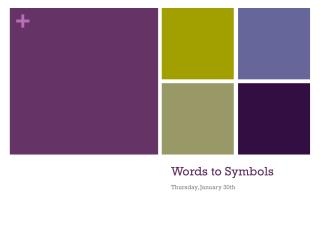DownloadDownload PresentationWords to Symbols

# Words to Symbols

Télécharger la présentation## Words to Symbols

- - - - - - - - - - - - - - - - - - - - - - - - - - - E N D - - - - - - - - - - - - - - - - - - - - - - - - - - -
##### Presentation Transcript

1. Words to Symbols Thursday, January 30th

2. Words to symbols Example: The tip of an iceberg is the part that you see sticking out of the water. Let’s call the height of that tip t. The height of the tip is just 1/7th of the height of the whole iceberg. Create an equation for the whole iceberg h.

3. Words to symbols Example: The tip of an iceberg is the part that you see sticking out of the water. Let’s call the height of that tip t. The height of the tip is just 1/7th of the height of the whole iceberg. Create an equation for the whole iceberg h. 1 7 t = h So h = …?

4. Words to symbols Example: The tip of an iceberg is the part that you see sticking out of the water. Let’s call the height of that tip t. The height of the tip is just 1/7th of the height of the whole iceberg. Create an equation for the whole iceberg h. 1 7 t = h So h = 7t

5. Words to symbols Which of the following equations represents this scenario: The number of scorpions eaten by Ada has increased by 2. • 2n • 2 + n • 2 – n • n – 2

6. Words to symbols Which of the following equations represents this scenario: The number of scorpions eaten by Ada has increased by 2. • 2n • 2 + n • 2 – n • n – 2

7. Words to symbols Which of the following equations represents this scenario: Victoria lets in a third of the goals that most goalies would let in. • 3n • n – 3 • n / 3 • 3 - n

8. Words to symbols Which of the following equations represents this scenario: Victoria lets in a third of the goals that most goalies would let in. • 3n • n – 3 • n / 3 • 3 - n

9. Words to symbols Which of the following equations represents this scenario: Calvin decreases the number of opponents in his video game by 2. • 2n • 2 + n • 2 – n • n – 2

10. Words to symbols Which of the following equations represents this scenario: Calvin decreases the number of opponents in his video game by 2. • 2n • 2 + n • 2 – n • n – 2

11. Words to symbols Which of the following equations represents this scenario: Kate is running twice as fast as her previous speed. • 2n • 2 + n • 2 – n • n – 2

12. Words to symbols Which of the following equations represents this scenario: Kate is running twice as fast as her previous speed. • 2n • 2 + n • 2 – n • n – 2

13. Try it! Academic class: • Page 8 #1 – 23 (odd numbered questions only), #25 with a partner • Page 9 #1 – 5 • Extra time: Page 2 Applied class: Geometry (page 5 – 7) • Page 8 #2 – 6

14. Geometry The length of a rectangular field is 3 times longer than the width, w. • Write an algebraic expression for the length, l. • Write an algebraic expression for the perimeter of the field (length of a fence around the field) • Write an algebraic expression for the area of the field

15. Rearranging Equations

16. z 2y x What might this represent?

17. z 2y x Example: x + z = 2y

18. z 2y x x = …

19. z 2y x x = 2y – z

20. z 2y x y = …

21. z 2y x + z 2 x y =

22. 3z = z = 2y 3z 2x y = 2y =

23. 3z = 2x + 2y z = 2y 3z 2x y = 2y =

24. 3z = 2x + 2y z = (2x + 2y) 3 2y 3z 2x y = 2y =

25. z = (2x + 2y) 3 3z = 2x + 2y 2y 3z 2x y = 2y = 3z – 2x

26. z = (2x + 2y) 3 3z = 2x + 2y 2y 3z 2x y = (3z – 2x) 2 2y = 3z – 2x

27. 2y = 3x + 4z Draw this!

28. 3x – z = 2y Draw this!

29. 3x – z = 2y Solve for 3x!

30. 3x – z = 2y Solve for x!

31. True or False? z = x + 2y + g3 x + 2y = 3z - g

32. Rearranging Equations Activity • In teams of two: • Cut out each of the equations and representative bars. • Glue 4 coloured bar graphs to a sheet of cardstock, leaving space underneath each. • Figure out which equations describe the bar graphs. Glue these underneath the correct graph. • Write one new equation using each each bar graph solving for a different variable.